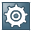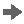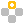Show TOC

###Special Depreciation MethodIn many countries, you are allowed to carry out special depreciations for your fixed assets. Usually, a certain percentage of the asset value can be depreciated in addition to the normal depreciation amount. The percentage allowed for the special depreciation, as well as the period over which you can carry out the special depreciation, are dependent on national legislation.Note

In SAP Business One, special depreciation is always carried out in the first period of the concession years. For more information, see the examples below.

End of the note.
##### Special Depreciation Method
 Displays Additional, which suggests that special depreciation is always calculated in addition to the standard depreciation amount.
 From the dropdown list, select a pool to which you want to assign the depreciation type. You must assign a special or manual depreciation type to a pool.
 Specify a depreciation type that is used for the regular automatic depreciation of an asset. You must specify a depreciation type whose method is not special depreciation.
 To compare different depreciation calculations, specify a second depreciation type here. The system calculates the depreciation for this depreciation type in parallel. The result of the alternative calculation is for reference only, and no bookings are carried out in the general ledger.RecommendationYou can specify the same depreciation type for the normal and alternative depreciation. This way, you can compare the asset depreciation with and without the special depreciation. End of the recommendation.
 Enter the number of years during which the special depreciation is legally permitted. You can enter integers only.
 If required, enter the percentage rate for calculating the maximum depreciation amount allowed in addition to the normal depreciation. National legislation specifies this value. The system calculates the maximum special depreciation amount as follows: (Acquisition and Production Costs – Salvage Value) * Maximum Percentage
 If required, enter the maximum depreciation amount allowed in addition to the normal depreciation. The maximum amount is an alternative to the maximum percentage.

To optimize depreciation from a tax point of view, you can split the concession period into multiple sub-periods and freely distribute the maximum percentage over these periods.

 Specify the number of years in each period. You can enter integers only.NoteThe total number of years you enter must not exceed the concession period. End of the note.
 Enter the annual percentage rate for the special depreciation calculation.NoteThe total percentage rate of all periods must not exceed the maximum percentage. End of the note.
 Enter the annual special depreciation amount.NoteThe total amount for all periods must not exceed the maximum amount. End of the note.Example

Asset X

Acquisition and Production Costs: 60,000 USD

Useful Life: 60 Months

Depreciation Type: Special Depreciation

Calculation Base: Yearly

Normal Depreciation: Depreciation Type 02 (Method = Straight Line; Calculation Base = Yearly; Calculation Method = Net Book Value/Remaining Life)

Capitalization Date: January 1st, 2010

Concession Period: 2 Years

Maximum Percentage: 30

Level

Number of Years

Annual Percentage

1

2

15

The system calculates the asset depreciation as follows:

• 2010–2011

The system first calculates the normal depreciation in 2010 and 2011 as follows:

2010: 60000 USD / 60 * 12 = 12000 USD

2011: (60000 USD – 12000 USD) / (60 – 12) * 12 = 12000 USD

Then, the special depreciation in each year is calculated as follows:

60000 USD * 15% = 9000 USD

Assuming your company's posting periods are months, SAP Business One carries out the special depreciation in the first month of 2010 and 2011, respectively.

• 2012–2014

The system calculates the depreciation from 2012 to 2014 as follows:Note

Within the concession period, the special depreciation amount does not affect the calculation of the net book value. However, after the concession period, the calculation of the net book value takes into account the special depreciation amount.

End of the note.
• 2012

(60000 USD – 12000 USD * 2 – 9000 USD * 2) / (60 – 12 * 2) * 12 = 6000 USD

• 2013

(60000 USD – 12000 USD * 2 – 9000 USD * 2 – 6000 USD) / (60 – 12 * 3) * 12 = 6000 USD

• 2014

60000 USD – 12000 USD * 2 – 9000 USD * 2 – 6000 USD * 2 = 6000 USD

Jan.

Feb.

Mar.

Apr.

May

Jun.

Jul.

Aug.

Sep.

Oct.

Nov.

Dec.

2010

10000

1000

1000

1000

1000

1000

1000

1000

1000

1000

1000

1000

2011

10000

1000

1000

1000

1000

1000

1000

1000

1000

1000

1000

1000

2012

500

500

500

500

500

500

500

500

500

500

500

500

2013

500

500

500

500

500

500

500

500

500

500

500

500

2014

500

500

500

500

500

500

500

500

500

500

500

500

End of the example.Example

Asset Y

Acquisition and Production Costs: 60,000 USD

Useful Life: 60 Months

Depreciation Type: Special Depreciation

Calculation Base: Yearly

Normal Depreciation: Depreciation Type 02 (Method = Straight Line; Calculation Base = Yearly; Calculation Method = Net Book Value/Remaining Life)

Capitalization Date: January 1st, 2010

Concession Period: 2 Years

Maximum Amount: 20,000 USD

Level

Number of Years

Amount

1

2

10000

The system calculates the asset depreciation as follows:

• 2010–2011

The system calculates the normal depreciation in 2010 and 2011 as follows:

2010: 60000 USD / 60 * 12 = 12000 USD

2011: (60000 USD – 12000 USD) / (60 – 12) * 12 = 12000 USD

The special depreciation in each year is 10,000 USD.

Assuming your company's posting periods are months, SAP Business One carries out the special depreciation in the first month of 2010 and 2011, respectively.

• 2012–2014

The system calculates the depreciation from 2012 to 2014 as follows:Note

Within the concession period, the special depreciation amount does not affect the calculation of the net book value. However, after the concession period, the calculation of the net book value takes into account the special depreciation amount.

End of the note.
• 2012

(60000 USD – 12000 USD * 2 – 10000 USD * 2) / (60 – 12 * 2) * 12 = 5333.33 USD

The system rounds 5,333.33 USD to 5,333 USD.

• 2013

(60000 USD – 12000 USD * 2 – 10000 USD * 2 – 5333 USD) / (60 – 12 * 3) * 12 = 5333.33 USD

The system rounds 5,333.33 USD to 5,333 USD.

• 2014

60000 USD – 12000 USD * 2 – 10000 USD * 2 – 5333 USD * 2 = 5334 USD

Jan.

Feb.

Mar.

Apr.

May

Jun.

Jul.

Aug.

Sep.

Oct.

Nov.

Dec.

2010

11000

1000

1000

1000

1000

1000

1000

1000

1000

1000

1000

1000

2011

11000

1000

1000

1000

1000

1000

1000

1000

1000

1000

1000

1000

2012

444.42

444.42

444.42

444.42

444.42

444.42

444.42

444.42

444.42

444.42

444.42

444.38

2013

444.42

444.42

444.42

444.42

444.42

444.42

444.42

444.42

444.42

444.42

444.42

444.38

2014

444.50

444.50

444.50

444.50

444.50

444.50

444.50

444.50

444.50

444.50

444.50

444.50

End of the example.